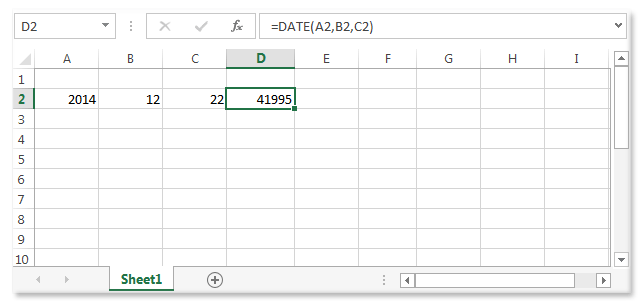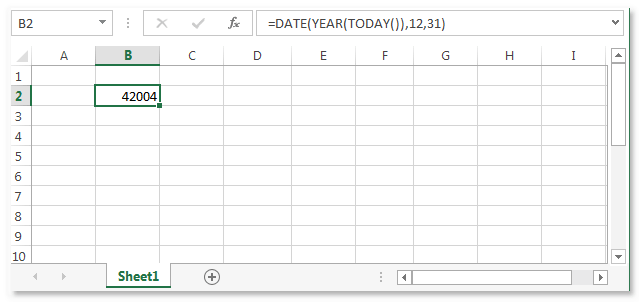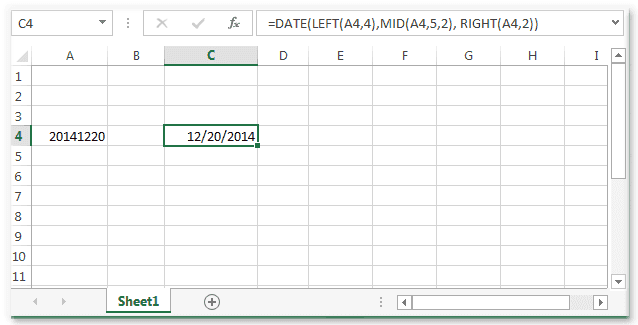# Excel DATE() function

## DATE() function

The DATE function is used to return the sequential serial number that represents a particular date.

Note : If the cell format was General before the function was entered, the result is formatted as a date instead of a number. To view the serial number or to change the formatting of the date change the cell format.

Version: Excel 2013

Syntax:

```DATE(year,month,day)
```
Name Description Data Type Required/
Optional
Year The value of the year argument can include one to four digits. By default, Microsoft Excel for Windows uses the 1900 date system. Number Required
Month A positive or negative integer representing the month of the year from 1 to 12 (January to December). Number Required
Day A positive or negative integer representing the day of the month from 1 to 31 Number Required

Important Points:

• If the year is between 0 (zero) and 1899 (inclusive), Excel adds that value to 1900 to calculate the year. For example, DATE(114,12,20) returns December 20, 2014 (1900+114).
• If the year is between 1900 and 9999 (inclusive), Excel uses that value as the year. For example, DATE(2014,12,20) returns December 20, 2014.
• If the year is less than 0 or is 10000 or greater, Excel returns the #NUM! error value.
• If the month is greater than 12, month adds that number of months to the first month in the year specified. For example, DATE(2014,20,12) returns the serial number representing August 12, 2015.
• If the month is less than 1, month subtracts the magnitude of that number of months, plus 1, from the first month in the year specified. For example, DATE(2014,-3,20) returns the serial number representing September 20, 2013.
• If the day is greater than the number of days in the month specified, day adds that number of days to the first day in the month. For example, DATE(2014,11,50) returns the serial number representing December 20, 2014.
• If the day is less than 1, day subtracts the magnitude the number of days, plus one, from the first day of the month, specified. For example, DATE(2014,1,-15) returns the serial number representing December 16, 2013.

Remarks:

Excel stores date as sequential serial numbers so that they can be used in calculations. January 1, 1900, is serial number 1, and January 1, 2010, is serial number 40179 because it is 40178 days after January 1, 1900.

Example - 1:

The example below shows the serial data or the date derived by using year as 2004 and month as 12 and day as 22.Example - 2:

The serial date for the last day of the current year.Example - 3:

The example below shows that, a formula that converts the text string in A4 (20141220) representing a date in a "YYYYMMDD" format to a date.Previous: Excel BETA.DIST() function
Next: Excel DATEVALUE() function

﻿